# Module 1 - Data Preparation

In this module, we’ll discuss how to prepare our sample data for time series analysis with NumXL.

Sample Data

Consider the daily adjusted Closing prices are adjusted for splits and dividends. closing prices for shares of Microsoft stock between 1/3/2000 and May 1st, 2009.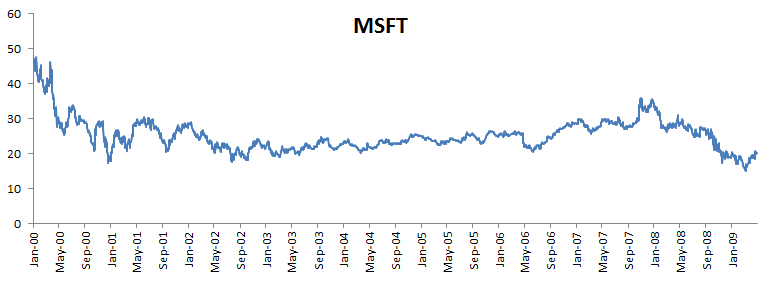## 1.1 Data Layout in Excel

Once you have your sample data, the most common time series layout method is to display the dates and values in adjacent columns in the same spreadsheet. Although the date component is not needed for modeling, it gives us a general idea about the chronological order of the values. All NumXL functions support two different chronological orders:

Ascending DescendingAscending: the first value corresponds to the earliest observation. NumXL assumes an ascending order by default unless otherwise specified.

Note: To make things simpler, you can create a defined name range in Excel for your input data. You can replace the range of cells with the defined name in all NumXL functions and wizards/forms.

## 1.2 Sampling

Once you have the ordered time series in your worksheet, you should examine the sampling assumptions.

A time-series data sample will generally contain observations that are equally spaced over time, where the value of each observation is available (i.e. there are no missing values).

For the MSFT daily closing price sample data, the observations are tacked to the end of each workday. In this case, the sample period is the trading day (not the calendar day), and the observations in the sample are equally spaced as a result.

Note: In the event, the sample data contains one or more missing values, a special treatment is required to impute their values. Refer to the "Missing Values" issue in the NumXL Tips & Hints archive online.

Once you have the ordered time series in your worksheet and have made a note of the sampling assumption, you should examine the data visually to ensure that it meets the important assumptions defined by econometric and time series theories:

1. Is the underlying process stable (Homogeneity)?
2. Do the variance and auto-covariance remain the same throughout the sample span (stationarity) ?
3. Do we have observations with unusual values?
4. Are the values of the observations well spread out?

## 1.3 Stationarity

For stationarity, we are mainly concerned with the stability of variances and covariance throughout the sample.

The stationarity assumption is pivotal for time series theories, so how do we check for it? Paradoxically, we start by testing for non-stationary conditions, primarily: (1) the presence of unit root (random walk) and/or (2) the presence of a deterministic trend. If we can’t find them, we may conclude that the data is stationary.

Let’s examine the plot for the original data for a deterministic trend or random walk (possibly with a drift).For the MSFT price time series, the data plot does not exhibit any trend and the series seems stationary.

Note: For more details on time series stationarity, refer to the "Stationarity" issue in the NumXL Tips & Hints archive online.

## 1.4 Homogeneity

Before we attempt to propose a model for a time series, we ought to verify that the underlying process did not undergo a structural change, at least during the span of the sample data.

What are structural changes? Structural changes are those events that permanently alter the statistical properties of the stochastic process. A structural change can be triggered by new changes in policies, passing new laws, or any major development (exogenous) during the span of the sample.

To examine for homogeneity (or the lack thereof), look over the data plot along with WMA and EWMA and try to identify any (permanent) changes in the mean, variance, or any signs of trend or random walk.

Furthermore, an analyst/investigator must bring rich prior knowledge and strong hypotheses about the underlying process structure to his interpretation of a data set.

In the plot below, we draw a 20-day equally-weighted moving average along with the original data.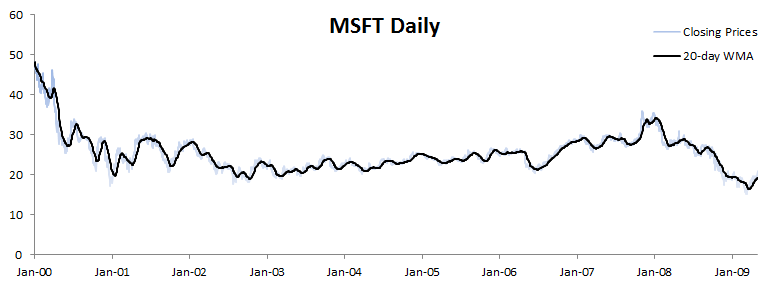Examining the sample data plot and the weighted-moving average (WMA), there is no evidence of a sudden permanent change in the underlying process mean.

Note: For more details on time series homogeneity, refer to the "Homogeneity" issue in the NumXL Tips & Hints archive online.

## 1.5 Outliers

An outlier is an observation that is numerically distant from the rest of the data. In other words, an outlier is one that appears to deviate markedly from other members of the sample in which it occurs.

The mere presence of outliers in our data may change the mean level in the uncontaminated time series, or it might suggest that the underlying distribution has fat-tails.

Outlier detection is a complex topic; for starters, we can examine the data plot visually. There are a few statistical methods to mark potential outliers, but it is your responsibility to verify, and to some extent explain their values.

Note: This is a quick overview of a very complex subject. For more details on time series outliers, refer to the "Outliers" issue in the NumXL Tips & Hints online.

A quick way to examine the outliers in the data is through the use of a Q1, Q3 quartile (i.e. IQR.).

$$\mathrm{LL}=\mathrm{Q1}-1.5\times\mathrm{IQR}$$ $$\mathrm{UL}=\mathrm{Q3}+1.5\times\mathrm{IQR}$$

In the plot below, the shaded region represents the values between the upper fence (UL) and the lower fence value (UL).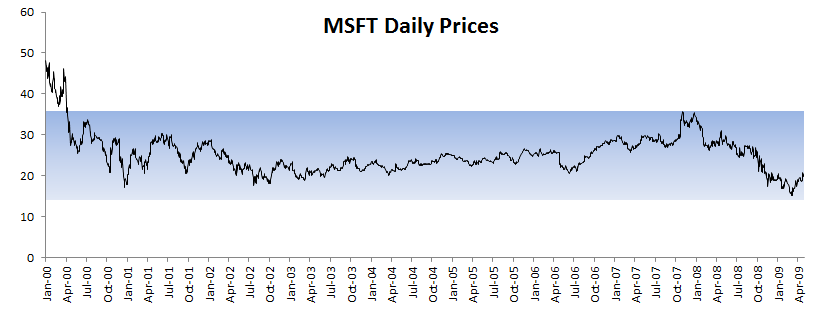One may argue that the values of the observations at the beginning of the sample are very high.

## 1.6 Concentration of Values

Occasionally, we face a time series in which values are naturally restricted to a given range. For example, binomial data are restricted between 0 and 1. Another example is company quarterly revenues, which are listed as positive integers within a wide range.

### Why should we care?

First, the time series model does not assume any bounds or limits on values that the time series can take, so using those models for a constrained data set may yield poor fitting/

Second, having a floor or a ceiling level in the data set affects the symmetry (or lack of skew) of the values around the mean. This phenomenon can also be difficult to capture using time series models.

Third, a data set whose values span several orders of magnitude can prove to be problematic for modeling and forecasting.

Finally, a relationship between the observation level and local variance may develop and, for the same reasons above, we’ll have to stabilize the variance before doing anything else.

To detect the issues associated with the concentration of values, we ask the following questions:

1. Is the volatility/variance changing in relation to the observation levels?
2. Are the data values capped or floor-leveled?
3. Does the distribution show a skew in either direction?

Assuming we have a concentration of values issue, what is next? We need to perform a data transformation on the input data.

Goal: we like the values of the observations to be distributed close to a normal distribution.

Let’s examine the distribution of the daily closing prices for MSFT shares. First, we plot the histogram and QQ-plot of daily closing prices: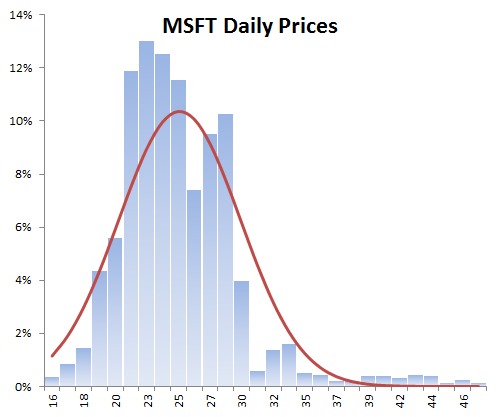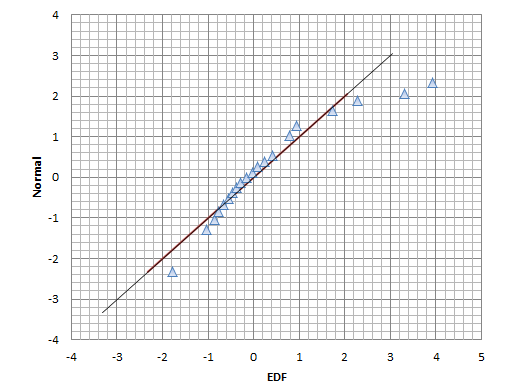Obviously, the data is far from normal, but the takeaway here is that 50% of the observations fall in a narrow range (22.18 – 27.61).

Next, let’s transform the values using the Box-Cox function. A Box-Cox transformation is a special form of power transformation and requires one input – lambda.

By optimizing the Box-Cox transformation for our sample data, we found that a zero value lambda (i.e. Log transformation) brings our data close to normality.

In the figure below, we plotted the histogram and QQ-plot for the log price.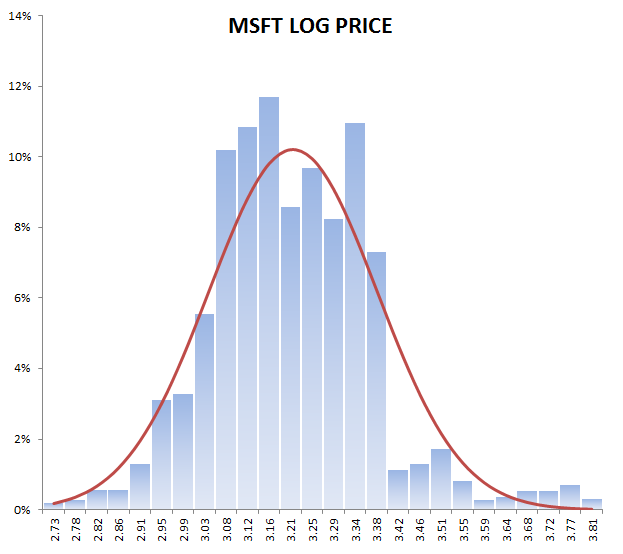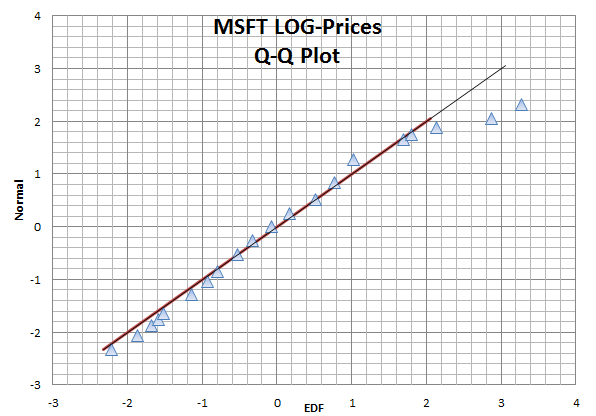The log (Box-Cox with zero-lambda) transformation improves the distribution of the values, especially at the right-tail.

## Conclusion

In this module, we discussed sample data layout in Excel, sampling assumptions of the data, and highlighted four (4) important issues to examine in our data before we conduct the analysis.

For the concentration of values, we used histograms and an empirical distribution function to show that 50% of values are concentrated in a narrow band. To bring the distribution close to the normal distribution, we used the Box-Cox transformation and optimized for lambda (i.e. Box-Cox parameter) and found that logarithmic (a special case of Box-Cox) is the best transformation. For the remainder of the user guide, we will use the log-transformation of the sample data to carry on the analysis.